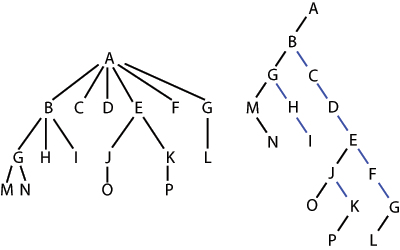## Chapter 6. Trees II

### 6.3 Binary Trees

A binary tree is an ordered tree in which every node has at most two children. A binary tree is proper if each internal node has two children.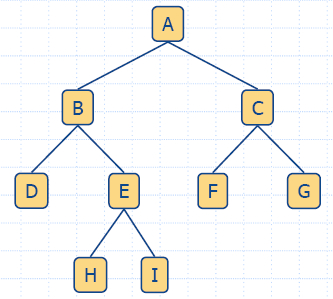A binary tree is a tree with the following properties: Each internal node has two children The children of a node are an ordered pair We call the children of an internal node left child and right child Alternative recursive definition: a binary tree is either a tree consisting of a single node, or a tree whose root has an ordered pair of children, each of which is a binary tree

Examples: arithmetic expression, decision tree

Arithmetic Expression TreeBinary tree associated with an arithmetic expression internal nodes: operators external nodes: operands Example: arithmetic expression tree for the expression (2 * (a −1) + (3 * b))

Decision Tree

• Binary tree associated with a decision process
• internal nodes: questions with yes/no answer
• external nodes: decisions
• Example: dining decision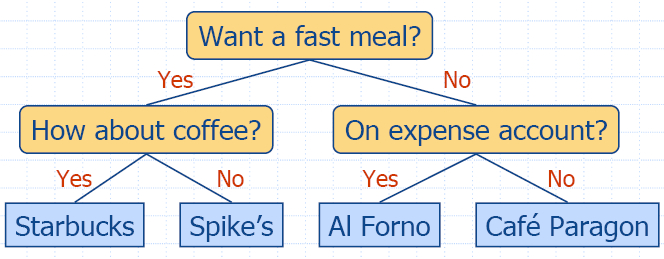• The BinaryTree ADT extends the Tree ADT, i.e., it inherits all the methods of the Tree ADT
• Position leftChild(Position p)
• Position rightChild(Position p)
• Position sibling(Position p)
• Update methods may be defined by data structures implementing the BinaryTree ADT

#### 6.3.2 A Binary Tree Interface

html-6.14a (InspectableBinaryTree)
html-6.14b (BinaryTree)

#### 6.3.3 Properties of Binary Trees

We denote the set of all nodes of a binary tree T, at the same depth d, as the level d of T.
Proposition 6.9: Let T be a proper binary tree with n nodes and let h denote the height of T. Then T has the following properties:
1. The number of external nodes e in T is: h + 1 e 2h
2. The number of internal nodes i in T is: h i 2h 1
3. The total number of nodes n in T is: 2h + 1 n 2h+1 1
4. The height h of T is: log(n + 1) 1 h (n 1)/2
Proposition 6.10: In a proper binary tree  T, the number of external nodes e is 1 more than the number of internal nodes i, i.e. e = i + 1.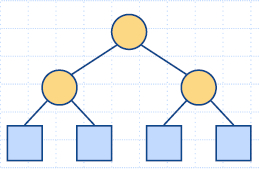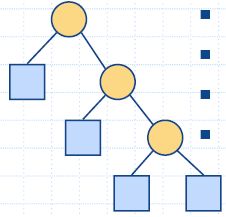Notation n number of nodes e number of external nodes i number of internal nodes h height Properties: e = i + 1 n = 2e − 1 h ≤ i h ≤ (n − 1)/2 e ≤ 2h h ≥ log2e h ≥ log2(n + 1)−1

Traversals of a Binary Tree

Preorder Traversal of a Binary Tree
`void binaryPreorderPrint(const Tree& T, const Position& v) { cout << v.element();		// print element  if (isInternal(v))		// visit children  {  cout << " ";     binaryPreorderPrint(T, T.leftChild(v));     binaryPreorderPrint(T, T.rightChild(v));  }}`
Postorder Traversal of a Binary Tree
`void binaryPostorderPrint(const Tree& T, const Position& v) { if (isInternal(v))    // visit children  { cout << " ";    binaryPostorderPrint(T, T.leftChild(v));    binaryPostorderPrint(T, T.rightChild(v));  }  cout << v.element(); // print element}`
Evaluating an Arithmetic Expression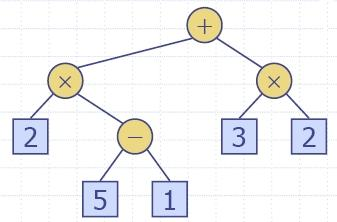Specialization of a postorder traversal recursive method returning the value of a subtree when visiting an internal node, combine the values of the subtrees O(n) time algorithm

 Algorithm evalExpr(v)       if isExternal (v)             return v.element ()       else           x ← evalExpr(leftChild (v))           y ← evalExpr(rightChild (v))           ◊ ← operator stored at v           return x ◊ y

Inorder Traversal of a Binary Tree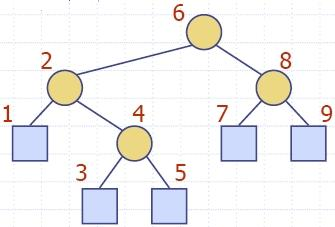In an inorder traversal a node is visited after its left subtree and before its right subtree Application: draw a binary tree x(v) = inorder rank of v y(v) = depth of v Visit "left to right"

 Algorithm inOrder(v)    if isInternal (v)        inOrder(leftChild (v))    visit(v)    if isInternal (v)       inOrder(rightChild (v))

`void binaryInorderPrint(const Tree& T, const Position& v) { if (isInternal(v))        // visit left child     binaryInorderPrint(T, T.leftChild(v));  cout << v.element();      // print element  if (isInternal(v))        // visit right child     binaryInorderPrint(T, T.rightChild(v));}`
Binary Search Trees

Binary search tree
is a binary tree so that each internal node v stores an element e, such that:
• the elements stored in the left subtree of v are less than or equal to e, and
• the elements stored in the right subtree of v are greater than or equal to e.
`Position searchBinaryTree(const Tree& T, const Position& v, const Object& e){ if (isInternal(v))    if (v.element() == e) return v;                 // found!    else if (v.element() < e)         searchBinaryTree(T, T.leftChild(v), e);    // search left subtree    else searchBinaryTree(T, T.rightChild(v), e);   // search right subtree  else return ...                                   // not found!}`
The time for searching in a binary tree T is proportional to the height of T, i.e. >= O(log n) and <= Omega(n)

A Unified Tree Traversal Framework

The Euler Tour Traversal of a Binary Tree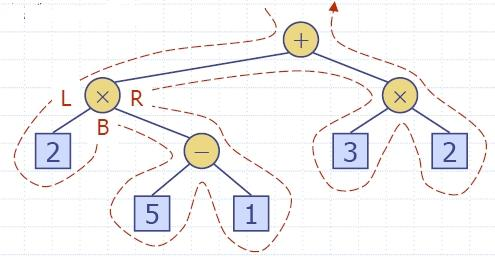Generic traversal of a binary tree Includes a special cases the preorder, postorder and inorder traversals Walk around the tree and visit each node three times: on the left (preorder) from below (inorder) on the right (postorder)

### 6.4 Data Structures for Representing Trees

#### 6.4.2 A Linked Structure for Binary Trees

• A node is represented by an object storing
• element
• link to the parent node
• link to the left child node
• link to the right child node
• Node objects implement the Position ADT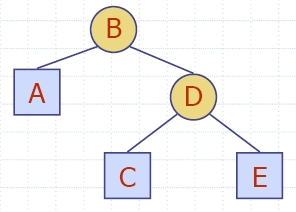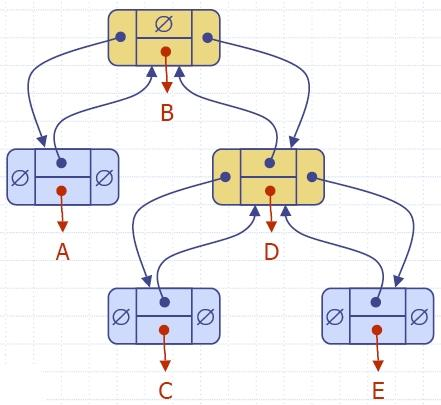Nodes and Positions in a Binary Tree
html-6.27 (Node)
html-6.28 (Position)

Binary Tree Update Functions

#### 6.4.3 A Linked Structure for General Trees

• A node is represented by an object storing
• element
• link to the parent node
• sequence of links to the children nodes
• Node objects implement the Position ADT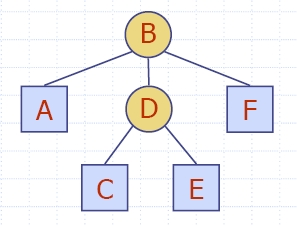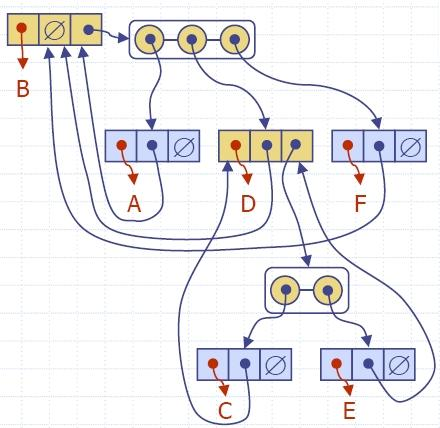#### 6.4.4 Representing General Trees with Binary Trees

A representation of a general (ordered) tree T  is obtained by transforming T  into a binary tree T '. The transformation is as follows:
• For each node u of T, there is an internal node u' of T ' associated with it.
• If u is an external node of T and does not have a sibling immediately following it, then the children of u' are external nodes.
• If u is an internal node of T and v is the first child of u in T, then v' is the left child of u' in T '.
• If node v has a sibling w immediately following it, then w' is the right child of v' in T '.